Next: Projection methods Up: METHODS FOR EXPLORATORY DATA Previous: Visualization of high-dimensional data

Clustering methods

The goal of clustering is to reduce the amount of data by categorizing or grouping similar data items together. Such grouping is pervasive in the way humans process information, and one of the motivations for using clustering algorithms is to provide automated tools to help in constructing categories or taxonomies [Jardine and Sibson, 1971, Sneath and Sokal, 1973]. The methods may also be used to minimize the effects of human factors in the process.

Clustering methods [Anderberg, 1973, Hartigan, 1975, Jain and Dubes, 1988, Jardine and Sibson, 1971, Sneath and Sokal, 1973, Tryon and Bailey, 1973] can be divided into two basic types: hierarchical and partitional clustering. Within each of the types there exists a wealth of subtypes and different algorithms for finding the clusters.

Hierarchical clustering proceeds successively by either merging smaller clusters into larger ones, or by splitting larger clusters. The clustering methods differ in the rule by which it is decided which two small clusters are merged or which large cluster is split. The end result of the algorithm is a tree of clusters called a dendrogram, which shows how the clusters are related. By cutting the dendrogram at a desired level a clustering of the data items into disjoint groups is obtained.

Partitional clustering, on the other hand, attempts to directly decompose the data set into a set of disjoint clusters. The criterion function that the clustering algorithm tries to minimize may emphasize the local structure of the data, as by assigning clusters to peaks in the probability density function, or the global structure. Typically the global criteria involve minimizing some measure of dissimilarity in the samples within each cluster, while maximizing the dissimilarity of different clusters.

A commonly used partitional clustering method, K-means clustering
[MacQueen, 1967], will be discussed in some detail since it is closely related to the SOM algorithm. In K-means clustering the criterion function is the average squared distance of the data items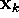from their nearest cluster centroids,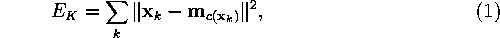where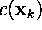is the index of the centroid that is closest to. One possible algorithm for minimizing the cost function begins by initializing a set of K cluster centroids denoted by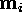,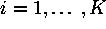. The positions of theare then adjusted iteratively by first assigning the data samples to the nearest clusters and then recomputing the centroids. The iteration is stopped when E does not change markedly any more. In an alternative algorithm each randomly chosen sample is considered in succession, and the nearest centroid is updated.

Equation 1 is also used to describe the objective of a related method, vector quantization [Gersho, 1979, Gray, 1984, Makhoul et al., 1985]. In vector quantization the goal is to minimize the average (squared) quantization error, the distance between a sample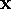and its representation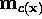. The algorithm for minimizing Equation 1 that was described above is actually a straightforward generalization of the algorithm proposed by Lloyd (1957) for minimizing the average quantization error in a one-dimensional setting.

A problem with the clustering methods is that the interpretation of the clusters may be difficult. Most clustering algorithms prefer certain cluster shapes, and the algorithms will always assign the data to clusters of such shapes even if there were no clusters in the data. Therefore, if the goal is not just to compress the data set but also to make inferences about its cluster structure, it is essential to analyze whether the data set exhibits a clustering tendency. The results of the cluster analysis need to be validated, as well. Jain and Dubes (1988) present methods for both purposes.

Another potential problem is that the choice of the number of clusters may be critical: quite different kinds of clusters may emerge when K is changed. Good initialization of the cluster centroids may also be crucial; some clusters may even be left empty if their centroids lie initially far from the distribution of data.

Clustering can be used to reduce the amount of data and to induce a categorization. In exploratory data analysis, however, the categories have only limited value as such. The clusters should be illustrated somehow to aid in understanding what they are like. For example in the case of the K-means algorithm the centroids that represent the clusters are still high-dimensional, and some additional illustration methods are needed for visualizing them.Next: Projection methods Up: METHODS FOR EXPLORATORY DATA Previous: Visualization of high-dimensional data